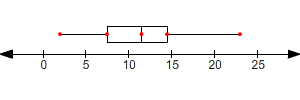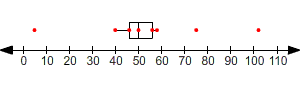# Box-and-Whisker Plots

To understand box-and-whisker plots, you have to understand medians and quartiles of a data set.

The median is the middle number of a set of data, or the average of the two middle numbers (if there are an even number of data points).

The median ( ${Q}_{2}$ ) divides the data set into two parts, the upper set and the lower set. The lower quartile ( ${Q}_{1}$ ) is the median of the lower half, and the upper quartile ( ${Q}_{3}$ ) is the median of the upper half.

Example:

Find ${Q}_{1}$ , ${Q}_{2}$ , and ${Q}_{3}$ for the following data set, and draw a box-and-whisker plot.

$\left\{2,6,7,8,8,11,12,13,14,15,22,23\right\}$

There are $12$ data points. The middle two are $11$ and $12$ . So the median, ${Q}_{2}$ , is $11.5$ .

The "lower half" of the data set is the set $\left\{2,6,7,8,8,11\right\}$ . The median here is $7.5$ . So ${Q}_{1}=7.5$ .

The "upper half" of the data set is the set $\left\{12,13,14,15,22,23\right\}$ . The median here is $14.5$ . So ${Q}_{3}=14.5$ .

A box-and-whisker plot displays the values ${Q}_{1}$ , ${Q}_{2}$ , and ${Q}_{3}$ , along with the extreme values of the data set ( $2$ and $23$ , in this case):A box & whisker plot shows a "box" with left edge at ${Q}_{1}$ , right edge at ${Q}_{3}$ , the "middle" of the box at ${Q}_{2}$ (the median) and the maximum and minimum as "whiskers".

Note that the plot divides the data into $4$ equal parts. The left whisker represents the bottom $25%$ of the data, the left half of the box represents the second $25%$ , the right half of the box represents the third $25%$ , and the right whisker represents the top $25%$ .

## Outliers

If a data value is very far away from the quartiles (either much less than ${Q}_{1}$ or much greater than ${Q}_{3}$ ), it is sometimes designated an outlier . Instead of being shown using the whiskers of the box-and-whisker plot, outliers are usually shown as separately plotted points.

The standard definition for an outlier is a number which is less than ${Q}_{1}$ or greater than ${Q}_{3}$ by more than $1.5$ times the interquartile range ( $\text{IQR}={Q}_{3}-{Q}_{1}$ ). That is, an outlier is any number less than ${Q}_{1}-\left(1.5×\text{IQR}\right)$ or greater than ${Q}_{3}+\left(1.5×\text{IQR}\right)$ .

Example:

Find ${Q}_{1}$ , ${Q}_{2}$ , and ${Q}_{3}$ for the following data set. Identify any outliers, and draw a box-and-whisker plot.

$\left\{5,40,42,46,48,49,50,50,52,53,55,56,58,75,102\right\}$

There are $15$ values, arranged in increasing order. So, ${Q}_{2}$ is the ${8}^{\text{th}}$ data point, $50$ .

${Q}_{1}$ is the ${4}^{\text{th}}$ data point, $46$ , and ${Q}_{3}$ is the ${12}^{\text{th}}$ data point, $56$ .

The interquartile range $\text{IQR}$ is ${Q}_{3}-{Q}_{1}$ or $56-47=10$ .

Now we need to find whether there are values less than ${Q}_{1}-\left(1.5×\text{IQR}\right)$ or greater than ${Q}_{3}+\left(1.5×\text{IQR}\right)$ .

${Q}_{1}-\left(1.5×\text{IQR}\right)=46-15=31$

${Q}_{3}+\left(1.5×\text{IQR}\right)=56+15=71$

Since $5$ is less than $31$ and $75$ and $102$ are greater than $71$ , there are $3$ outliers.

The box-and-whisker plot is as shown. Note that $40$ and $58$ are shown as the ends of the whiskers, with the outliers plotted separately.